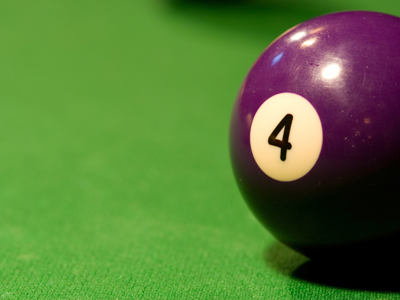To calculate one quarter of a number, we divide by 4.

# Fractions (Year 4)

As you go through KS2 Maths, you'll discover more about fractions. In Year Four, you'll learn about smaller fractions like twentieths and how to calculate them.

Fractions can be written in different ways. For example, 10 twentieths is the same as 10/20, 1/2, or 2/4. When you calculate fractions, divide the number by the bottom one and then multiply by the top one. For instance, to find four twentieths of forty, divide 40 by 20 (equals 2), then multiply by 4 to get the answer 8.

Check out our 'How To Teach My Child About Fractions' article for helpful tips on explaining parts of numbers.

1.
Which represents one eighth?
14
18
120
15
18 is the same as 1 divided by 8
2.
Which fraction is greater than 12?
14
510
26
1120
1020 is equivalent to 12 so 1120 must be more
3.
What is one tenth of 30?
3
6
10
20
To calculate one tenth we divide by 10
4.
Which of these fractions is equivalent to one quarter?
28
13
36
25
One eighth is half of one quarter so two eighths is equivalent to one quarter
5.
Which of these fractions is equivalent to 34?
23
610
46
68
6 ÷ 2 = 3 and 8 ÷ 2 = 4 so 34 is the same as 68
6.
Which of these is NOT equivalent to 12?
24
36
23
48
23 is more than 12
7.
Which fraction is the greatest?
410
56
23
1120
56 is 16 less than 1
8.
If we divide a number by 3 the result will be?
14
110
16
13
9 ÷ 3 = 3 so 13 of 9 = 3
9.
Which is the smallest of these fractions?
14
18
120
16
If the numbers above the line are equal then the fraction with the highest number below the line is smaller
10.
How do we calculate one quarter of a number?
Divide by 2
Multiply by 4
Divide by 4
Multiply by 2
16 ÷ 4 = 4 so 14 of 16 is 4